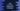# Can Enum implements interfaces in Java## Can Enum implements interfaces in Java:

Enum is a special data type in Java. Enum is used to define a list of constants. These are final static by default. The name of a enum field is written in upper case.

Interfaces holds methods with empty bodies. A class can implement interfaces and implement these methods.

In Java, an enum can also implement interfaces. In this post, we will learn how enum implements interfaces with examples.

## Java example to illustrate Enum implementing interface:

Let’s take a look at the below example :

``````interface ICalculator {
int getResult(int a, int b);
}

enum CalculatorType implements ICalculator {
PLUS {
@Override
public int getResult(int a, int b) {
return a + b;
}
},

MINUS {
@Override
public int getResult(int a, int b) {
return a - b;
}
},

MULTIPLY {
@Override
public int getResult(int a, int b) {
return a * b;
}
},

DIVIDE {
@Override
public int getResult(int a, int b) {
return a / b;
}
}
}

class Calculator {
private int a, b;
CalculatorType type;

public Calculator(int a, int b, CalculatorType type) {
this.a = a;
this.b = b;
this.type = type;
}

public int getResult() {
return type.getResult(a, b);
}
}

class Main {
public static void main(String[] args) {
Calculator calculator1 = new Calculator(10, 2, CalculatorType.PLUS);
Calculator calculator2 = new Calculator(10, 2, CalculatorType.MINUS);
Calculator calculator3 = new Calculator(10, 2, CalculatorType.MULTIPLY);
Calculator calculator4 = new Calculator(10, 2, CalculatorType.DIVIDE);

System.out.println("10 + 2 : " + calculator1.getResult());
System.out.println("10 - 2 : " + calculator2.getResult());
System.out.println("10 * 2 : " + calculator3.getResult());
System.out.println("10/2 : " + calculator4.getResult());
}
}``````

### Explanation:

This program shows how we can create a calculator using enum. Here,

• ICalculator is an interface that has only one method getResult.
• CalculatorType enum implements ICalculator. So, for each enum value, it overriding getResult, and getResult returns different result for each enum.
• Calculator is a class that holds two integers and one CalculatorType enum.
• We are creating different Calculator objects with different enum values. The getResult of Calculator class calls the getResult for each enum separately and returns the value based on the enum stored in the variable type.

### Output:

If you run this program, it will print the below output:

``````10 + 2 : 12
10 - 2 : 8
10 * 2 : 20
10/2 : 5``````